Select Page

# CBSE Maths 12 Science Continuity and Differentiability Answers for MCQ in English

CBSE Maths 12 Science Continuity and Differentiability Answers for MCQ in English to enable students to get Answers in a narrative video format for the specific question.

Expert Teacher provides CBSE Continuity and Differentiability Maths 12 Science Answers for MCQ through Video Answers in English language. This video solution will be useful for students to understand how to write an answer in exam in order to score more marks. This teacher uses a narrative style for a question from Continuity and Differentiability not only to explain the proper method of answering question, but deriving right answer too.

Please find the question below and view the Answer in a narrative video format.

Question:

## Similar Questions from CBSE, 12th Science, Maths, Continuity and Differentiability

Question 1 : Differentiate the function w.r.t.x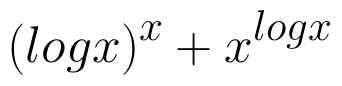. (View Answer Video)

Question 2 : Findfor the function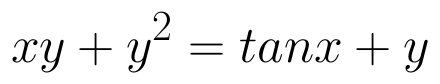. (View Answer Video)

Question 3 : Using the fact that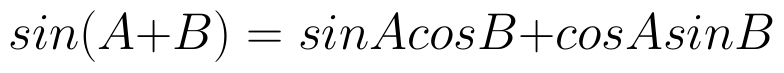and the differentiation, obtain the sum formula for cosines. (View Answer Video)

Question 4 : Differentiate the function w.r.t.x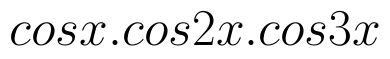. (View Answer Video)

Question 5 : Differentiate the function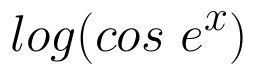with respect to x. (View Answer Video)

### Differential Equations

Question 1 : Solve the differential equation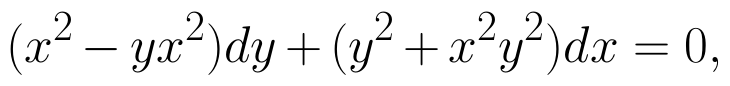given that y = 1 when x = 1. (View Answer Video)

Question 2 : Find the particular solution of the differential equation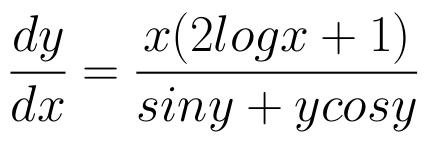given that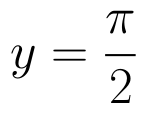where x = 1. (View Answer Video)

Question 3 : Find the particular solution of the differential equation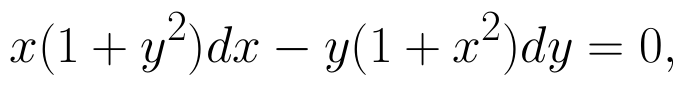given that y = 1 when x = 0.  (View Answer Video)

Question 4 : Solve the differential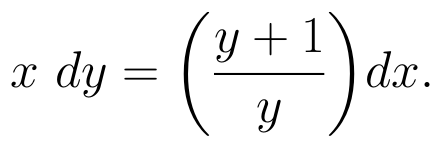(View Answer Video)

Question 5 : Solve the differential equation: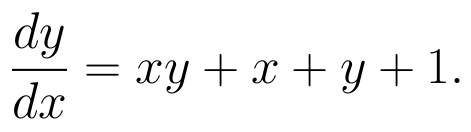(View Answer Video)

### Probability

Question 1 : There are three coins. One is a two-headed coin (having head on both faces), another is a biased coin that comes up heads 75% of the times and third is also a biased coin that comes up tails 40% of the times. One of the three coins is chosen at random and tossed, and it shows heads. What is the probability that it was the two-headed coin?  (View Answer Video)

Question 2 : If P(A)=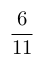, P(B)=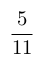and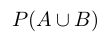=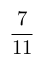, find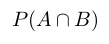. (View Answer Video)

Question 3 : An experiment succeeds thrice as often as it fails. Find the probability that in the next trials, there will be atleast 3 successes.    (View Answer Video)

Question 4 : A bag contains 4 red and 4 black balls, another bag contains 2 red and 6 black balls. One of the two bags is selected at random and two balls are drawn at random (without replacement) from the bag which are both found to be red. Find the probability that the balls are drawn from the first bag.    (View Answer Video)

Question 5 : A die is thrown 6 times. If 'getting an odd number' is a 'success', what is the probability of obtaining:
atleast 5 successes?